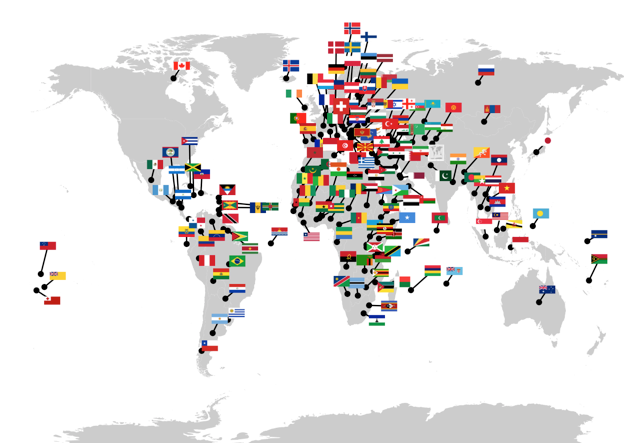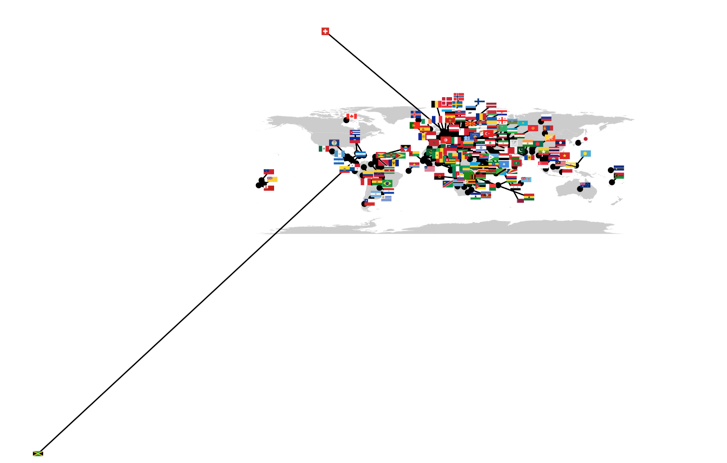ggrepel with maps

ggrepel is a package for R that build on ggplot an uses the new ggproto OO system for extending ggplot to provide a few new geom’s for placing text and labels. Its great. I’ve been working on some maps and was hoping I could take the same principles used in ggrepel to add images to a ggplot. In short, I couldn’t find a way that would let you use the ggproto system (unless there is a way to store grobs within data.frames that I don’t know about) but you can call ggrepel directly to find non-overlapping points for arbitrary boxes, and you can add plots, images etc to a grid grapchic using the annotation_custom function of ggplot.

My current results can be found on Rpubs and as a gist Below is a very quick overview of the code.

Flagmap#ggrepelled flagmap#

The core idea is to use ggrepel::repel_boxes() to get new coordinates and then plot those coords.

force = 1e-6, maxiter = 20000) {

......

moved <- ggrepel:::repel_boxes(data_points=as.matrix(posdf),
boxes = boxmatrix,
xlim=xlim,
ylim=ylim,
force=force,
maxiter=maxiter)

finaldf <- cbind(posdf, moved)
names(finaldf) <- c("x1","y1","x2","y2")
return(finaldf)
}

# this can them be used to add a plot
# where grob is an ImageGrob of a PNG file
gg <- gg + annotation_custom(grob,
xmin = long - xdiff,
xmax = long + xdiff,
ymin = lat - ydiff,
ymax = lat + ydiff)
return(gg)

# add a bunch of the files to the  Map